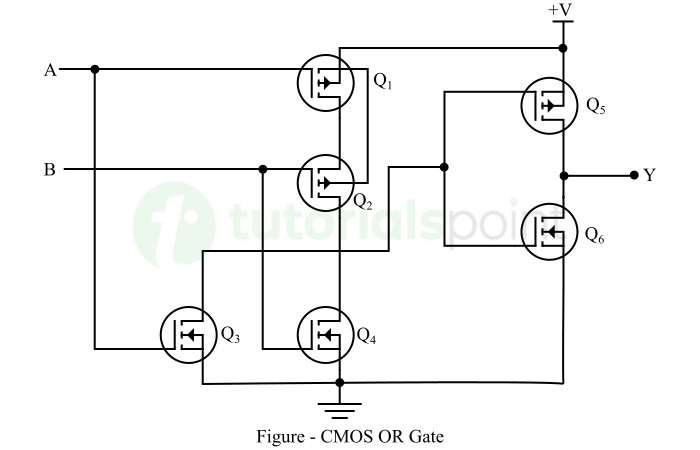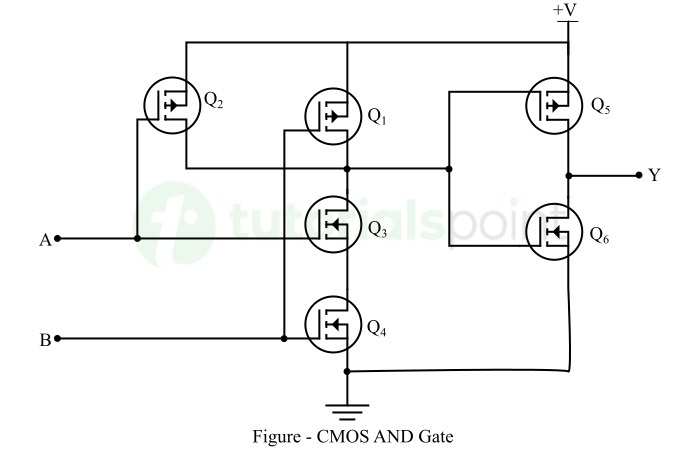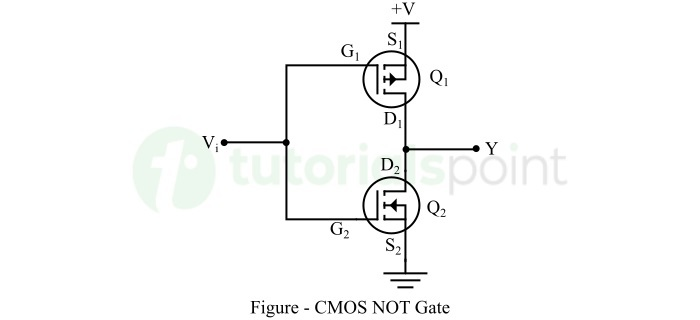# Basic CMOS Logic Gates

## What is a Logic Gate?

In digital circuits, a logic gate is a fundamental element of the digital circuit or system, that performs a specific logical operation. A logic gates typically has one or more inputs and only one output. The output of the logic gate is related to the inputs based on a certain logic.

Some commonly used logic gates are: AND Gate, OR Gate, NOT Gate, NAND Gate, and NOR Gate.

## What is CMOS Technology?

CMOS, Complementary Metal Oxide Semiconductor, is a predominant technology used for manufacturing integrated circuits (ICs). This technology is widely used for manufacturing a variety of digital electronic components like microprocessors, sensors, logic gates, and more.

CMOS technology employs both NMOS (N-Channel Metal Oxide Semiconductor) and PMOS (P-Channel Metal Oxide Semiconductor) logic to implement different types of digital functions.

CMOS technology is widely being used in realizing digital logic gates due to its key advantages, such as fast switching speed, low power consumption, high voltage range, high noise margins, etc.

## Basic CMOS Logic Gates

Let us now discuss the basic CMOS logic gates in detail.

### CMOS OR Gate

The OR gate is a basic logic gate in digital electronics. OR gates produce a high or logic 1 output when any of its inputs is high, and it produces a low or logic 0 output when all of its inputs are low.

The truth table of a two-input OR gate is given below.

Inputs Output
A B Y = A+B
0 0 0
0 1 1
1 0 1
1 1 1

The implementation of a two input OR gate in CMOS logic is shown in the following figure.Here, we have combined multiple CMOS inverters together to build a CMOS OR gate. In the circuit diagram, the CMOS OR gate consists of a PMOS transistor and an NMOS transistor, which are joined in parallel fashion between the power supply voltage (V) and the output.

In the above circuit, when one or both inputs (A and B) are high (logic 1), the corresponding PMOS and NMOS transistors switch in a way that the current flows from the output to ground, causes a low voltage (logic 0) at the output terminal.

When both inputs are low (logic 0), the corresponding CMOS transistors switch in a way that the output is connected to the supply voltage, causing a high voltage (logic 1) at the output terminal.

### CMOS AND Gate

The AND gate is a basic logic gate used in a variety of digital logic circuits. AND gate produces a high or logic 1 output only when all of its inputs are high, it produces a low or logic 0 output when any of its inputs is low.

The truth table of the two-input AND gate is given below.

Inputs Output
A B Y = AB
0 0 0
0 1 0
1 0 0
1 1 1

The implementation of AND gate using CMOS technology is shown in the following figure.Here, to implement the CMOS AND gate, we have connected multiple CMOS inverters together. In the CMOS AND gate circuit, PMOS and NMOS transistors are used to build it. These transistors are connected in series between supply voltage (V) and the output (Y), and in parallel between the output and ground respectively.

In the case of CMOS AND gate shown in the above figure, when both inputs are high (logic 1), the CMOS transistors switch in such a way that the output is connected to the power supply voltage (V), producing a high (logic 1) output.

When any of its inputs is low, the CMOS transistors in the circuit switch such that the current flows from power supply to the ground, making output low (logic 0).

### CMOS NOT Gate

A NOT gate is also a basic logic gate used in digital logic circuits. The NOT has a single input and a single output. The output of the NOT gate is high or logic 1 when its input is low or logic 0, and its output is low or logic 0 when its input is high or logic 1.

In CMOS logic gates, the CMOS NOT gate is the simplest CMOS logic gate. It is also called CMOS Inverter Gate.

The truth table of the NOT gate is given below.

Input Output
A Y = A’
0 1
1 0

The implementation of a NOT gate in CMOS technology is shown in the following figure.Here, the input is applied to the gate terminal of the two CMOS transistor, and the output is connected to their drain terminals.

When a positive voltage pulse (logic 1) is applied to the input Vi, the CMOS transistor Q1 is off and the CMOS transistor Q2 is on. Therefore, the output voltage will be at ground voltage, i.e. logic 0.

When the ground voltage (logic 0) is applied to the input Vi, the CMOS transistor Q1 is on and the CMOS transistor Q2 is off. Thus, the output will be closed to +V, i.e. logic 1.

## Conclusion

In this tutorial, we discussed in detail the implementation basic logic gates, i.e. AND gate, OR gate, and NOT gate in CMOS (Complementary Metal-Oxide Semiconductor) technology. The major advantages of CMOS logics include low output impedance, high input impedance, low power requirement. Due to all these benefits, the CMOS logic gates are widely used in many applications like arithmetic operations, control operations, data processing, and many other digital applications.

Updated on: 26-Jul-2023

923 Views